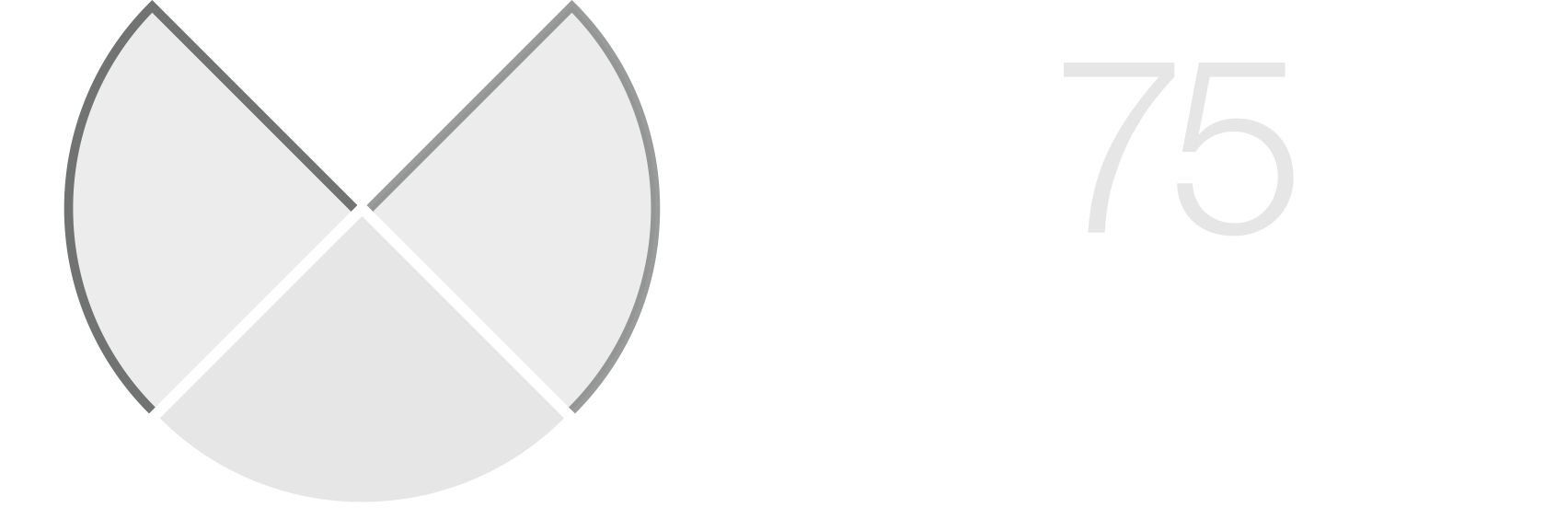﻿ MISANU
National Institute of the Republic of SerbiaPROGRAM

 ODELJENJE ZA MATEMATIKUMATEMATIČKOG INSTITUTA SANU

PROGRAM ZA JUN 2016.

Utorak, 14.06.2016. u 11:00, Sala 301f, MI SANU, Kneza Mihaila 36
Petar Pavešić, Fakulteta za matematiko in fiziko, Ljubljana
COMPLEXITY OF THE FORWARD KINEMATIC MAP
Given a robot or, more generally, a mechanical system with movable joints, the forward kinematic map determines the pose (position and orientation) of the end-effector as a function of the joint parameters. A typical forward kinematic map is therefore a smooth map $f:C\rightarrow W$, where the configuration space $C$ is a subspace of a product of circles (for revolute joints) and intervals (for sliding joints), and the working space W is a subspace of $R^3\times SO(3)$ (position and orientation of the end-effector). For an initial state of joint parameters $c$ in $C$ and a desired pose $w$ in $W$ one must determine a path in $C$ starting at $c$ and ending at some joint configuration $c'$ that yields the pose $w$ (i.e.\ $f(c')=w$). The topological complexity of f measures the difficulty of finding robust solutions to that problem - i.e.\ finding paths in $C$ that depend continuously on the initial data $(c,w)$. In the talk we are going to present a mathematical framework for the problem and describe some basic results and examples.

Utorak, 14.06.2016. u 11:45, Sala 301f, MI SANU, Kneza Mihaila 36
Neža Mramor-Kosta, Fakulteta za Računalništvo in informatiko, Ljubljana
DISCRETE MORSE THEORY AND DATA
In the last two decades since it was introduced, discrete Morse theory has proven to be an extremely useful tool in topological data analysis. We will first give an overview of the theory and its main results. Examples of applications to data from different domains, demonstrating how discrete Morse theoretical techniques can be used to extract useful information from data, as well as aid in solving problems like classification, clustering and prediction, will be presented.

Odeljenje za matematiku je opsti matematicki seminar namenjen sirokoj publici. Predavanja su prilagodjena matematicarima i onima koji zele da to postanu.

Zoran Petric, Odeljenje za matematiku Matematickog instituta SANU

﻿